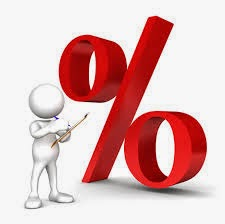[Career][grids]

## Personality

[Personality Development][twocolumns]

## Amazing Facts

[Amazing Facts][grids]

## Motivational

[Motivational Story][twocolumns]If the value of something is decreased by x % and again decreased by y % then resulting effect in percent
Total change = {-x – y + (-x)*(-y)/100}
Example :
The Salary of a worker is first decreased by 10% and thereafter decreased by 5%. What is the overall change in percent.
Solution:
overall change = – 10 – 5+ (-10)(-5)/100 = -14.5 % (decreased)
use (+) sign for increment and (-) sign for decrement.
Now, this is just the formula but its application are endless in the question of percentage , for the questions like-
1. If both the lengths (%) and breadth(%) of a rectangle are changed find the change in area(%) ?
2. If the speed and time of a vehicle are changed, find the change in distance ?
Similarly in all those questions where two quantities are multiplied to produce third one
rate * time = cost
efficiency * time = work
average * no. of elements = total value
rate * quantity = price (expenditure)

If the value of something is increased by x % and again increased by y % then resulting effect in percent
Total change = {x+y+x*y/100}
Example :
The Salary of a worker is first increased by 10% and thereafter increased by 5%. What is the overall change in percent.
Solution:
overall change = + 10 + 5 + (+10)(+5)/100 = +15.5 % (increased)
use (+) sign for increment and (-) sign for decrement.

If the value of something is increased by x % then decreased by y % then resulting effect in percent
Total change = {+x- y+ (x*(-y)/100)}
Example :
The Salary of a worker is first increased by 10% and thereafter decreased by 5%. What is the overall change in percent.
Solution:
overall change = + 10 – 5 +(10)(-5)/100 = +4.5 % (increased)

use (+) sign for increment and (-) sign for decrement.